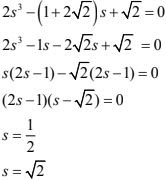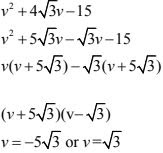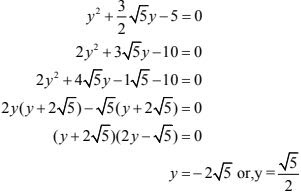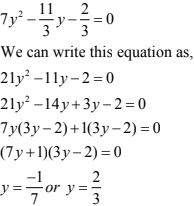# Chapter 2 Polynomials NCERT Exemplar Solutions Exercise 2.3 Class 10 MathsChapter Name NCERT Maths Exemplar Solutions for Chapter 2 Polynomials Exercise 2.3 Book Name NCERT Exemplar for Class 10 Maths Other Exercises Exercise 2.1Exercise 2.2Exercise 2.4 Related Study NCERT Solutions for Class 10 Maths

### Exercise 2.3 Solutions

Find the zeroes of the following polynomials by factorization method.
1. 4x2 – 3x - 1
2. 3x2  + 4x - 4
3. 5t2 + 12t + 7
4. t3 - 2t2 - 15t
5. 2x2 + 7x/2 + 3/4
6. 4x2 + 5√2x - 3
7. 2s3 - (1 + 2√2)s + √2
8. v2 + 4√3 v - 15
9. y2 + 3/2 √5 y - 5
10. 7y2 - 11y/3 - 2/3

Solution

1. 4x2 - 3x - 1
By splitting the middle term,
4x2 - 4x + 1x - 1
Now, taking out the common factors,
4x(x - 1) + 1 (x - 1)
= (4x + 1)(x - 1
The zeroes are,
4x + 1 = 0
⇒ 4x = - 1
⇒ x = -1/4
Also,
(x - 1) = 0
⇒ x = 1
Therefore, zeroes are -1/4 and.

2. 3x2 + 4x - 4

Solution

3x2 + 4x - 4
By splitting the middle term, we get,
3x2 + 6x - 2x - 4
= 3x(x + 2) - 2(x + 2)
= (x + 2)(3x - 2)
Either,
x +2 = 0
⇒ x = - 2
⇒ 3x - 2 =  0
⇒ 3x = 2
⇒ x = 2/3
Therefore, zeroes are 2/3 and -2.

3. 5t2 + 12t + 7

Solution

5t2 + 12t + 7
By splitting the middle term, we get,
5t2 + 5t + 7t + 7
= 5t(t + 1) + 7(t + 1)
= (t + 1)(5t + 7)
So, the zeroes, are,
t + 1 = 0
⇒ t = -1
⇒ 5t + 7 = 0
⇒ 5t = -7
⇒ t = -7/5
So, the zeroes are -7/5 and -1

4. t3 - 2t2 - 15t

Solution

t3 - 2t2 - 15t
t(t2 - 2t - 15)
Splitting the middle term of the equation t2 - 2t - 15, we get,
t(t2 - 5t + 3t - 15)
= t(t(t- 5) + 3 (t - 5)
= t(t + 3)(t- 5)
The zeroes are,
t + 3 = 0
⇒ t = -3
t - 5 = 0
⇒ t = 5
So, zeroes are 0, 5 and -3.

5. 2x2 + 7x/2 + 3/4

Solution

2x2 + 7x/2 + 3/4
We can write this equation as,
8x2 + 14x + 3
Now, splitting the middle term, we get,
8x2 + 12x + 2x + 3
= 4x(2x + 3) + 1 (2x + 3)
= (4x + 1)(2x + 3)
The zeroes are,
4x + 1 = 0
⇒ x = -1/4
⇒ 2x + 3 = 0
⇒ x = -3/2
Therefore, zeroes are -1/4 and -3/2.

6. 4x2 + 5√2x - 3

Solution

By splitting middle term, we get,
4x2 + 5√2x - 3
= 4x2 + 6√2x - √2x - 3
= 2√2x(√2x + 3) - 1(√2x + 3)
= (2√2x - 1)(√2x + 3)
Therefore,
x = 1/2√2
or,
x = -3/√2

7. 2s3 - (1 + 2√2)s + √2

Solution

By splitting middle term, we get,8. v2 + 4√3 v - 15

Solution

By splitting middle term, we get,9.  y2 + 3/2 √5 y - 5

Solution

By splitting middle term, we get,10. 7y2 - 11y/3 - 2/3

Solution

By splitting middle term, we get,